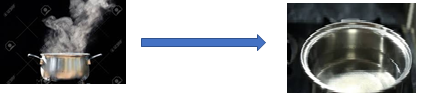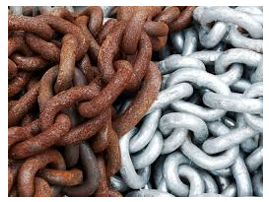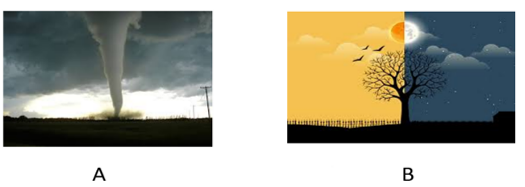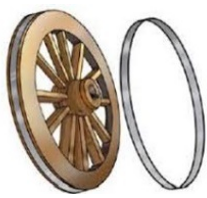# Extra Questions for Class 6 Science Chapter 6 Changes Around us

In this page we have Extra Questions for Class 6 Science Chapter 6 Changes Around us . Hope you like them and do not forget to like , social share and comment at the end of the page.

Question 1
(i)list two changes that occur without active interference of human beings.
(ii)Can you obtain wood from saw dust?
(iii)Why condensation is a physical change?
(iv)Ravi folded a sheet of paper to make a paper-boat. Comment on the nature of change that took place
(v)Name a change which occurs on heating but reversible and one which is irreversible.

Question 2
CLASSIFY THE FOLLOWING AS PHYSICAL And CHEMICAL CHANGE
(i)Pulling of iron spring
(ii)Breaking of glass beaker
(iii)Baking cake
(iv)Ploughing of field
(v)Blowing of balloon

Question 3
DEFINE THE FOLLOWING TERM
(i)Change
(ii)Exothermic reaction
(iii)Evaporation
(iv)Expansion
(v)Condensation

Question 4
ANSWER THE FOLLOWING QUESTIONS IN BRIEF
(i)What is reversible change? give two examples
(ii)Sumita broke a vase made of Plaster of Paris. Since she knew POP is present in dust form and water is added to it to solidify it, so she made a dust of the broken pieces and mixed it water and tried to make another vase. Will she be successful? Give reason
(iii)Differentiate between physical & chemical changes.
(iv)Give four examples of irreversible change
(v)To walk through a waterlogged area, you usually shorten the length of your dress by folding it. Can it be reversed?

Question 5(i)Name the process that leads to the formation of the second picture.
(ii)Define the above process.
(iii)Name the process which is opposite to the above process.
(iv)What will happen to the water if it is kept below 0o C?

Question 6(i)What is this picture showing?
(ii)How & What kind of change takes place to form the left side of the image from the right side of the image?
(iii)What is the classification of the change?

Question 7(i)Which of the figure is a non-periodic change? Why?
(ii)List two example of a non-periodic change.
(iii)List two examples of periodic changes.

Question 8(i)What is the above picture showing?
(ii)What is the normal size of the metal rim?
(iii)Name the process that helps the metal rim to fit on the wooden wheel.
(iv)Why & when cold water is added during the process?

• Notes
• Assignments & NCERT Solutions### Practice Question

Question 1 What is $\frac {1}{2} + \frac {3}{4}$ ?
A)$\frac {5}{4}$
B)$\frac {1}{4}$
C)$1$
D)$\frac {4}{5}$
Question 2 Pinhole camera produces an ?
A)An erect and small image
B)an Inverted and small image
C)An inverted and enlarged image
D)None of the above